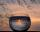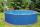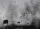The coil

How many ropes (the diameter 8 mm) fit on the coil (threads are wrapped close together) The coil has dimension: the inner diameter 400mm, the outside diameter 800mm and the length of the coil is 470mm

Result

x =  2768.529 m

Solution:Leave us a comment of example and its solution (i.e. if it is still somewhat unclear...):Be the first to comment!To solve this example are needed these knowledge from mathematics:

Do you want to convert area units? Do you want to convert length units? Do you know the volume and unit volume, and want to convert volume units?

Next similar examples:

1. Tin with oilTin with oil has the shape of a rotating cylinder whose height is equal to the diameter of its base. Canned surface is 1884 cm2. Calculate how many liters of oil is in the tin.
2. ContainerThe container has a cylindrical shape the base diameter 0.8 m and the area of the base is equal to the area of the wall. How many liters of water can we pour into the container?
3. Holidays - on poolChildren's tickets to the swimming pool stands x € for an adult is € 2 more expensive. There was m children in the swimming pool and adults three times less. How many euros make treasurer for pool entry?
4. Water wellDrilled well has a depth 20 meters and 0.1 meters radius. How many liters of water can fit into the well?
5. RainHow many mm of water rained the roof space 75 m2 if the empty barrel with a radius of 8 dm and height 1.2 m filled to 75% its capacity? :-)
6. Conserving waterCalculate how many euros are spent annually on unnecessary domestic hot water, which cools during the night in pipeline. Residential house has 129 m of hot water pipelines 5/8" and the hot water has a price of 7 Eur/m3.
7. PotCalculate the height of 3 liter pot with shape cylinder with a diameter of 10 cm.
8. Height as diameter of baseThe rotary cylinder has a height equal to the base diameter and the surface of 471 cm2. Calculate the volume of a cylinder.
9. Volume and surfaceCalculate the volume and surface area of the cylinder when the cylinder height and base diameter is in a ratio of 3:4 and the area of the cylinder jacket is 24 dm2.
10. Tank diameterA cylindrical tank has a volume of 60 hectoliters and is 2.5 meters deep. Calculate the tank diameter.
11. Cylindrical tankCylindrical tank holds 600hl water and is deep 2.5 m. Calculate the diameter of the cylinder.
12. Tank and waterCylindrical tank were poured with 3.5 liters of water. If tank base diameter is 3 dm, how height is water level in?
13. Garden poolMr. Novak fill garden pool (cylinder; diameter of 200 cm) with 31.4 hl of water. What is the depth of the pool when the water level is 10 cm below the upper edge of the pool?
14. Theorem proveWe want to prove the sentence: If the natural number n is divisible by six, then n is divisible by three. From what assumption we started?
15. An oilAn oil drum is cut in half. One half is used as a water trough. Use the dimensions; length 82cm, width 56cm to estimate the capacity of the water trough in liters.
16. The cylinder baseThe cylinder with a base of 8 dm2 has a volume of 120 liters. From a cylinder fully filled with water, 40 liters of water was removed. At what height from the bottom /with precision to dm/ is the water level?
17. WaterIn the garden with an area of 8 ares rain 40hl of water. To what heights leveled water?